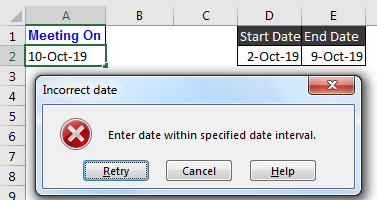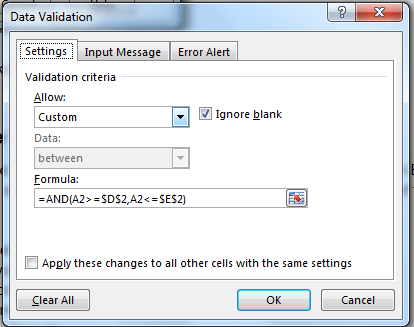# Excel Data Validation Between Dates

Sometimes we want users to pic dates from specified dates only. Irregular dates makes it hard to analyse data effectively and increase work overhead. In this article, we will learn how to put data validation for specified date only and make users enter dates only from that time interval.Let’s see an example to make things clear.
Example: Put Date Validation in Excel
Here I have an specified date interval in cell D2 and E2. In D2, start date is 2-Oct-19 and in E2, end date is 9-Oct-19. In cell A2 user will enter a meeting date. The criteria is that date can not be out of the above specified time interval.
To put date validation in Excel, follow these steps:

1. Select cell/s, where you want to put validation.
2. Go to Data->Validation. You can use keyboard shortcut ALT>A>V>V.
3. In allow, select custom.
4. In Formula., write this formula
5. =AND(A2>=\$D\$2,A2<=\$E\$2)6. In error alert, write this line in Error message box. “Enter date within specified date interval.”
7. Hit Ok. The work is done.

Now try to write any date which is before or after the given date in D2 and E2. Excel will not accept the input.
As you change the dates in start date and end date, the validation will change. There will be no need to change data validation.
But if you want to hardcode the dates then write this formula in formula input box of data validation.

=AND(A2>=DATE(2019,10,2),A2<=DATE(2019,10,2))

This will make the start date and end date fixed. You can copy A2 and paste special for data validation only to apply on other cells.
How it works?
Well, here we are using AND function to check if user is entering the correct data.

AND(A2>=\$D\$2,A2<=\$E\$2): Here, excel first checks the first criteria. If value in A2 is greater than or equal to value in D2, it return TRUE else FALSE.

Next excel checks the second criteria that if value in A2 is less than or equal to value in E2. If it is, than it returns TRUE else FALSE.

If either of the criteria returns FALSE, input is rejected.

So yeah guys, this how you put date validation in excel. Let me know if you have any doubts regarding this article or any other excel/VBA topic. The comments section is all yours.

Popular Articles:
The VLOOKUP Function in Excel

COUNTIF in Excel 2016

How to Use SUMIF Function in Excel

Terms and Conditions of use

The applications/code on this site are distributed as is and without warranties or liability. In no event shall the owner of the copyrights, or the authors of the applications/code be liable for any loss of profit, any problems or any damage resulting from the use or evaluation of the applications/code.